python中的类属性和实例属性

https://www.cnblogs.com/scolia/p/5582268.html

class Test(object):
name = 'scolia'

a = Test()
print Test.name  # 通过类进行访问
print a.name    # 通过实例进行访问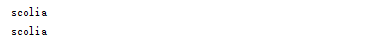class Test(object):
name = 'scolia'

a = Test()
Test.name = 'scolia good'   # 通过类进行修改
print Test.name
print a.name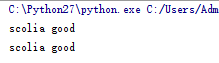class Test(object):
name = 'scolia'

a = Test()
a.name = 'scolia good'  # 通过实例进行修改
print Test.name
print a.name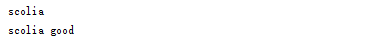如果用一张图来表示的话：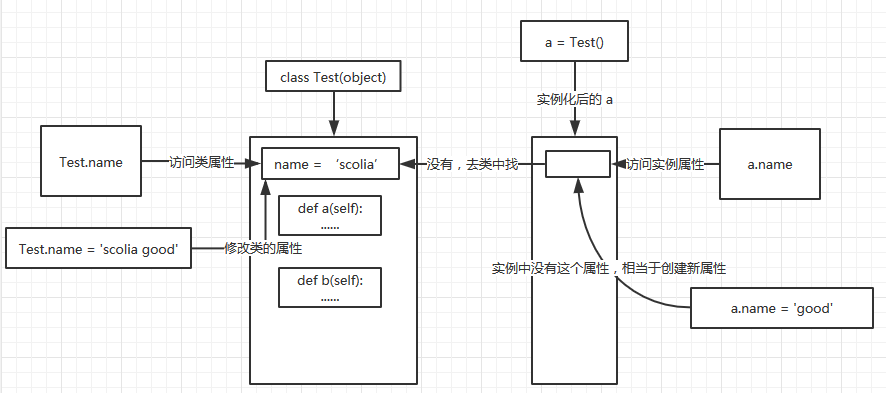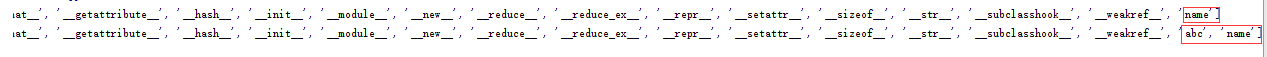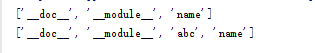__module__ 表示这个类来自哪个模块，我们在主文件中写的类，其值应该为 ‘__main__’，在其他模块中的类，其值就为模块名。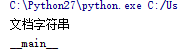__dict__

class Test:
"""文档字符串"""
name = 'scolia'

a = Test()
a.name = 'good'
print Test.__dict__
print a.__dict__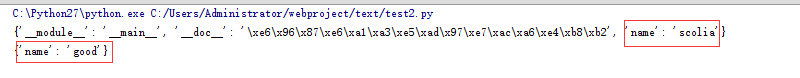__class__

class Test:
pass

a = Test()
print a.__class__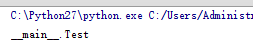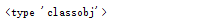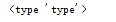b = a.__class__()
print b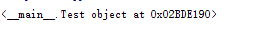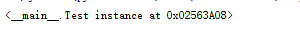class Test:
list1 = []

a = Test()
a.list1.append(123)     # 同通过实例修改类中的列表
print Test.list1
print a.list1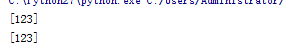a.list1 =    # 显式的创建一个新对象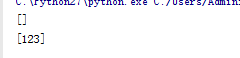class Test:
pass

def fangfa():
print '我是某个实例的方法'

a = Test()
b = Test()
a.abc = fangfa  # 特意添加一个方法
a.abc()
b.abc()     # b 没有这个方法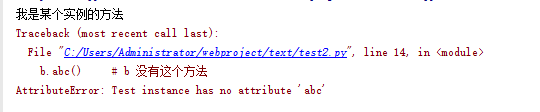class Test:
pass

def fangfa(self):   # self 代表是实例方法，只能由实例调用
print '我是方法'

Test.abc = fangfa
a = Test()
a.abc()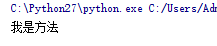字段私有化：

class Test:
__name = 'scolia'   # 私有字段

def a(self):
print Test.__name   #内部还需要用类来方问

a = Test()
a.a()
print Test.__name   # 在外部使用类来访问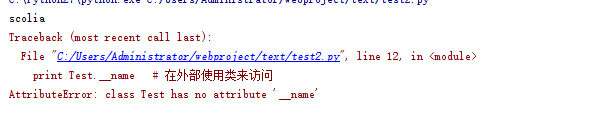print a.__name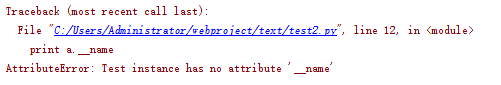print Test._Test__name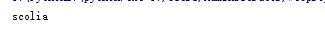class Test:
def __init__(self):
self.__name = 'scolia'   # 实例的私有字段

def a(self):
print self.__name   # 只能有内部方法能访问

a = Test()
a.a()
print a.__name  # 试图通过实例访问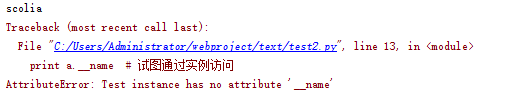print a._Test__name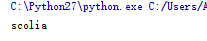总结：公用字段可以通过类对象，实例对象，类里面的方法进行访问。

而私有字段则一般通过类里面的方法进行访问。

一般不建议强制访问私有字段。

觉得文章有用就打赏一下文章作者

微信扫一扫打赏